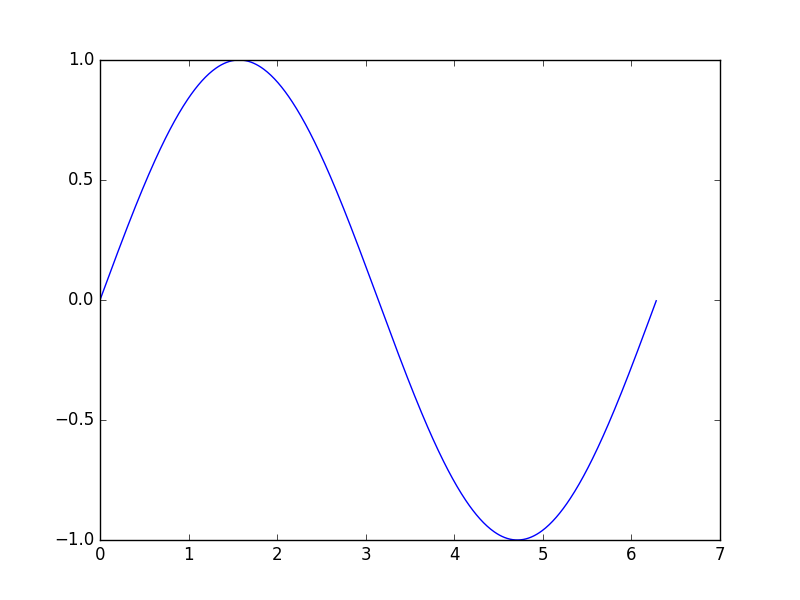# Animation 动画

## 定义方程 ¶

from matplotlib import pyplot as plt
from matplotlib import animation
import numpy as np
fig, ax = plt.subplots()


x = np.arange(0, 2*np.pi, 0.01)
line, = ax.plot(x, np.sin(x))def animate(i):
line.set_ydata(np.sin(x + i/10.0))
return line,


def init():
line.set_ydata(np.sin(x))
return line,


## 参数设置 ¶

1. fig 进行动画绘制的figure
2. func 自定义动画函数，即传入刚定义的函数animate
3. frames 动画长度，一次循环包含的帧数
4. init_func 自定义开始帧，即传入刚定义的函数init
5. interval 更新频率，以ms计
6. blit 选择更新所有点，还是仅更新产生变化的点。应选择True，但mac用户请选择False，否则无法显示动画
ani = animation.FuncAnimation(fig=fig,
func=animate,
frames=100,
init_func=init,
interval=20,
blit=False)


plt.show()


ani.save('basic_animation.mp4', fps=30, extra_args=['-vcodec', 'libx264'])